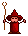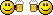###Author Topic: Rope physics  (Read 4142 times)

####r0ber7

• Prof. Inline
••• Posts: 552##### Rope physics
« on: 2013-Oct-22 »
In an attempt to create more interesting enemies for my game, I decided to figure out how ropes work. Not only could I use that code to, well, hang ropes around the place, I could also make snakes move around. It would also make creating endbosses more interesting and less time-consuming.

Anyway, here's the code. You can download the entire project as a zip file from here.

Code: (glbasic) [Select]
`// --------------------------------- //// Project: Ropes// Start: Tuesday, October 22, 2013// IDE Version: 10.283// Robert van Engelen// www.driewielerdigital.com// IDENTITYGLOBAL rope AS p_ropeGLOBAL mx, my, b1, b2MOUSESTATE mx, my, b1, b2rope.init( 20, mx, my)WHILE 1 MOUSESTATE mx, my, b1, b2 rope.play( mx, my) rope.draw()WEND// TYPETYPE p_rope// STATEstr1_x[] // pointsstr1_y[]str2_x[]str2_y[]vec_x1 // vectorsvec_x2vec_y1vec_y2at_x1 // points to attach both sides of rope toat_y1at_x2at_y2segments_n = 10gravity = 0.0txt\$ // text to print// BEHAVIOURFUNCTION init : n1, px, py, grav1=1.7, attach_x1=0, attach_y1=0, attach_x2=0, attach_y2=0self.segments_n = n1self.gravity = grav1LOCAL iLOCAL n = self.segments_nDIM self.str1_x[n+1]DIM self.str1_y[n+1]DIM self.str2_x[n+1]DIM self.str2_y[n+1]// initialize pointsFOR i=0 TO n-1 self.str1_x[i] = px self.str1_y[i] = py //INC self.str1_x[i], 0 INC self.str1_y[i], i*1 NEXTself.at_x1 = attach_x1self.at_y1 = attach_y1self.at_x2 = attach_x2self.at_y2 = attach_y2ENDFUNCTIONFUNCTION play : px, pyLOCAL i, n=self.segments_n, normal_length = 3LOCAL vec_x1, vec_x2, vec_y1, vec_y2, mag1, mag2, ext1, ext2, xv, yv// set the new top x and yself.str1_x = pxself.str1_y = pyFOR i=0 TO n-1STDOUT "I: "+i+"\n"IF i > 0 vec_x1 = self.str1_x[i-1] - self.str1_x[i] vec_y1 = self.str1_y[i-1] - self.str1_y[i] mag1 = SQR( POW(vec_x1, 2) + POW(vec_y1, 2) ) // get the length between two points ext1 = mag1 - normal_length STDOUT "ext1: "+ext1+" = "+mag1+"-"+normal_length+"\n" ENDIFvec_x2 = self.str2_x[i+1] - self.str2_x[i]vec_y2 = self.str2_y[i+1] - self.str2_y[i]mag2 = SQR( POW(vec_x2, 2) + POW(vec_y2, 2) ) // get the length between two pointsext2 = mag2 - normal_lengthSTDOUT "ext2: "+ext2+" = "+mag2+"-"+normal_length+"\n" //          xv = (X_Vector1 / Magnitude1 * Extension1) + (X_Vector2 / Magnitude2 * Extension2)//          yv = (Y_Vector1 / Magnitude1 * Extension1) + (Y_Vector2 / Magnitude2 * Extension2) + Gravityxv = ( vec_x1 / mag1 * ext1 ) + ( vec_x2 / mag2 * ext2 )yv = ( vec_y1 / mag1 * ext1 ) + ( vec_y2 / mag2 * ext2 ) + self.gravityIF vec_x1 = 0 //OR vec_x2 = 0 xv = 0ENDIFIF vec_y1 = 0 OR vec_y2 = 0 yv = 0ENDIFSTDOUT "xv: "+xv+"=("+vec_x1+"/"+mag1+"*"+ext1+"), yv:"+yv+"\n" self.str2_x[i] = self.str1_x[i] + ( xv*0.5 )self.str2_y[i] = self.str1_y[i] + ( yv*0.5 )NEXTFOR i=0 TO n-1 self.str1_x[i] = self.str2_x[i] self.str1_y[i] = self.str2_y[i]NEXTself.str1_x[n-1] = self.str1_x[n-2]self.str1_y[n-1] = self.str1_y[n-2]ENDFUNCTION// draw stuffFUNCTION draw :LOCAL n=self.segments_nALPHAMODE -0.6FOR i=0 TO n-2 DRAWRECT self.str1_x[i]+96, self.str1_y[i], 8, 8, RGB(255,0,0) DRAWRECT self.str2_x[i]+98, self.str2_y[i], 4, 4, RGB(255,255,0)NEXTSHOWSCREENENDFUNCTIONENDTYPE`

####mentalthink

• Prof. Inline
••• Posts: 3375
• Integrated Brain##### Re: Rope physics
« Reply #1 on: 2013-Oct-22 »
Very interesting and fun, I played a bit with the code, perhaps in 3D with vertex selection can create a nice FX...
Thanks for sharing the work.